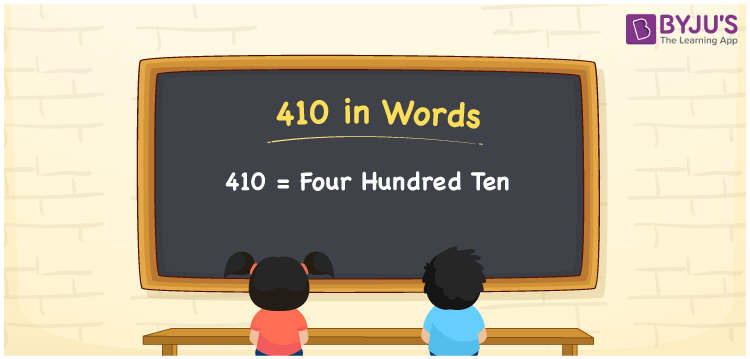# 410 in words

410 in words is written as Four Hundred and Ten. 410 represents the count or value. The article on Counting Numbers can give you an idea about count or counting. The number 410 is a 3 digit number that is used in expressions related to money, days, distance, length, weight and so on. Let us consider an example for 410. “I have Four Hundred and Ten contacts in my mobile”. Another example is “The capacity of an overhead tank is Four Hundred and Ten liters.”

 410 in words Four Hundred and Ten Four Hundred and Ten in Numbers 410

## 410 in English Words## How to Write 410 in Words?

We can convert 410 to words using a place value chart. The number 410 has 3 digits, so let’s make a chart that shows the place value up to 3 digits.

 Hundreds Tens Ones 4 1 0

Thus, we can write the expanded form as:

4 × Hundred + 1 × Ten + 0 × One

= 4 × 100 + 1 × 10 + 0 × 1

= 410

= Four Hundred and Ten.

410 is the natural number that is succeeded by 409 and preceded by 411.

410 in words – Four Hundred and Ten.

Is 410 an odd number? – No.

Is 410 an even number? – Yes.

Is 410 a perfect square number? – No.

Is 410 a perfect cube number? – No.

Is 410 a prime number? – No.

Is 410 a composite number? – Yes.

## Solved Example

1. Write the number 410 in expanded form

Solution: 4 × 100 + 1 × 10 + 0 × 1

We can write 410 = 400 + 10 + 0

= 4 × 100 + 1 × 10 + 0 × 1

## Frequently Asked Questions on 410 in words

Q1

### How to write the number 410 in words?

410 in words is written as Four Hundred and Ten.
Q2

### State True or False. 410 is divisible by 3?

False. 410 is not divisible by 3.
Q3

### Is 410 a perfect square number?

No. 410 is not a perfect square number.